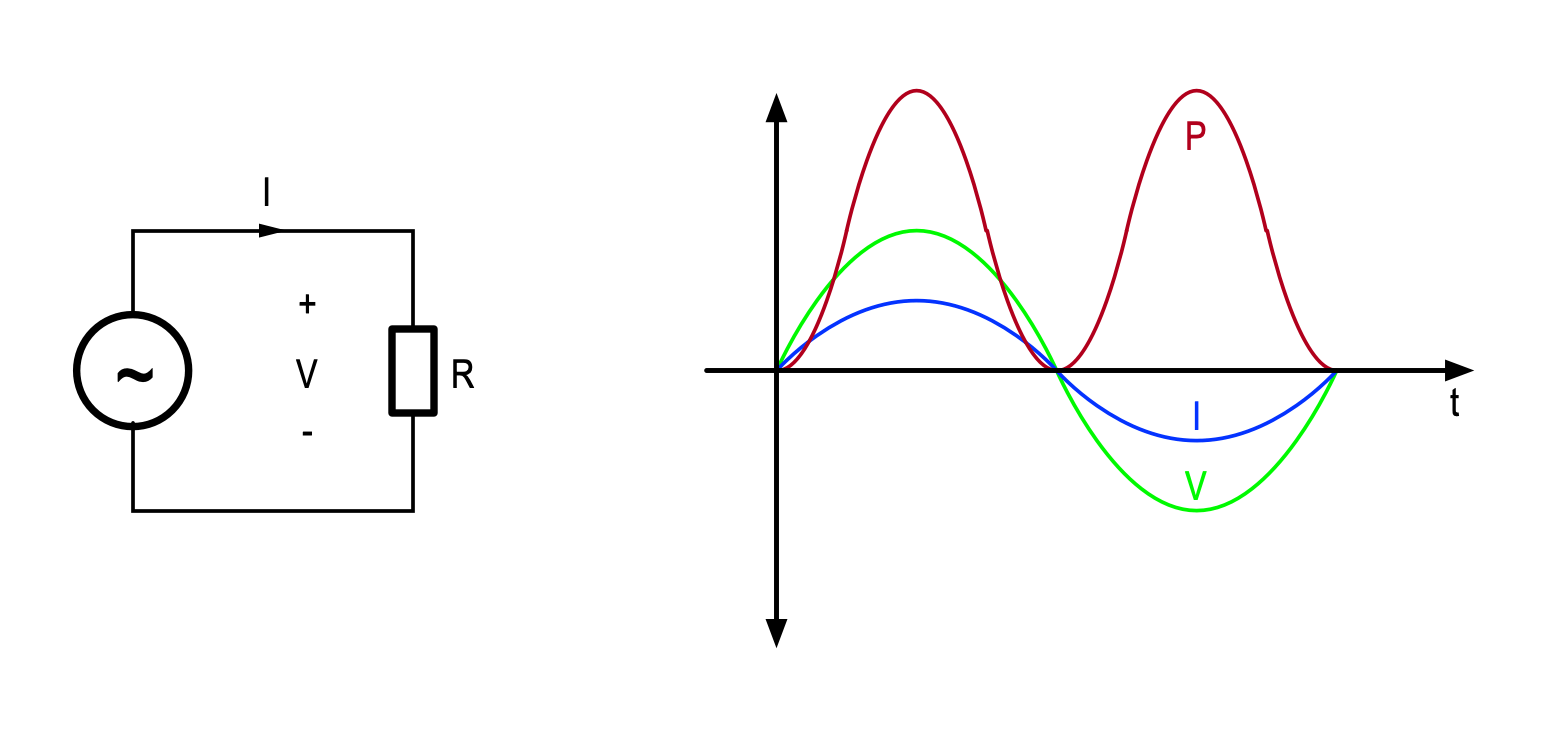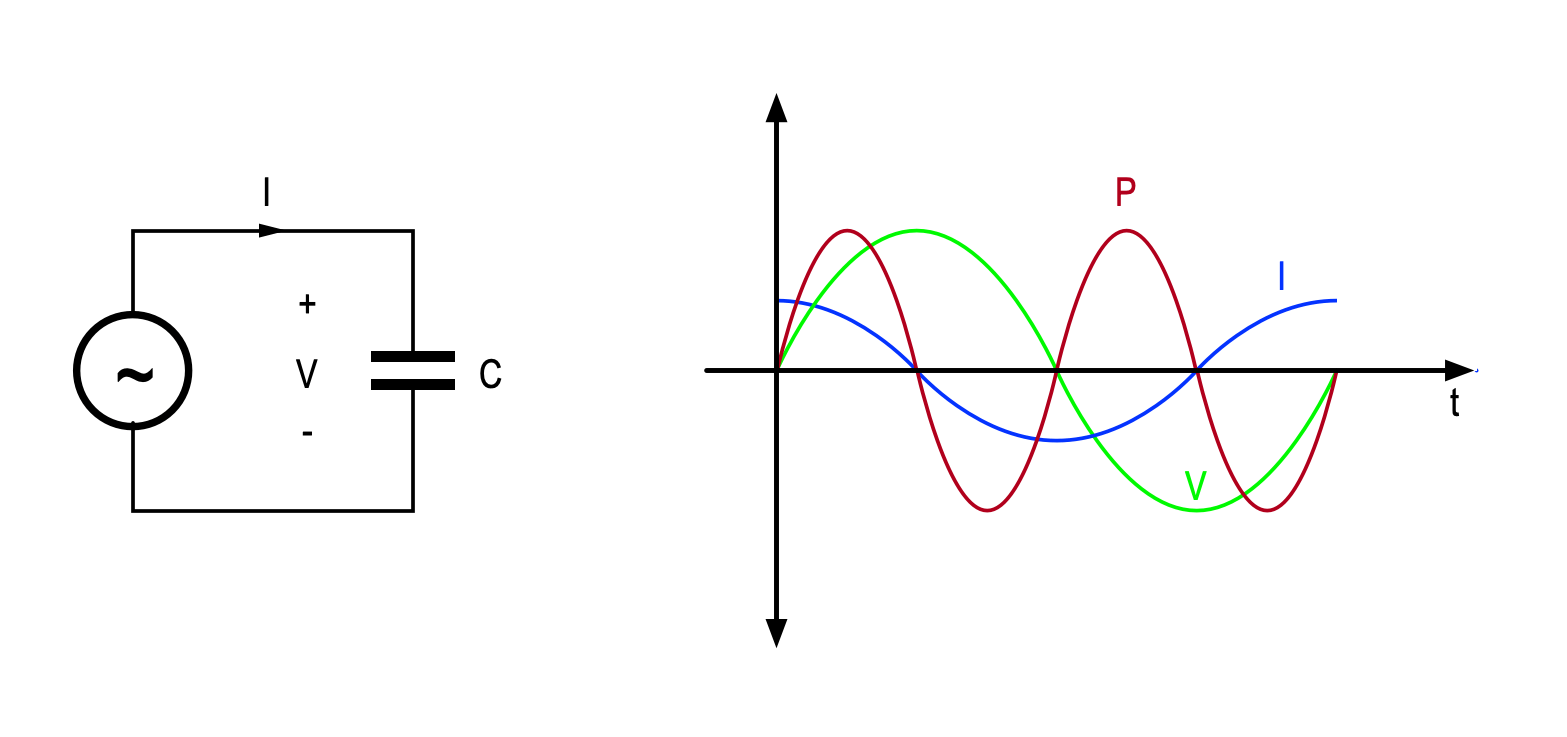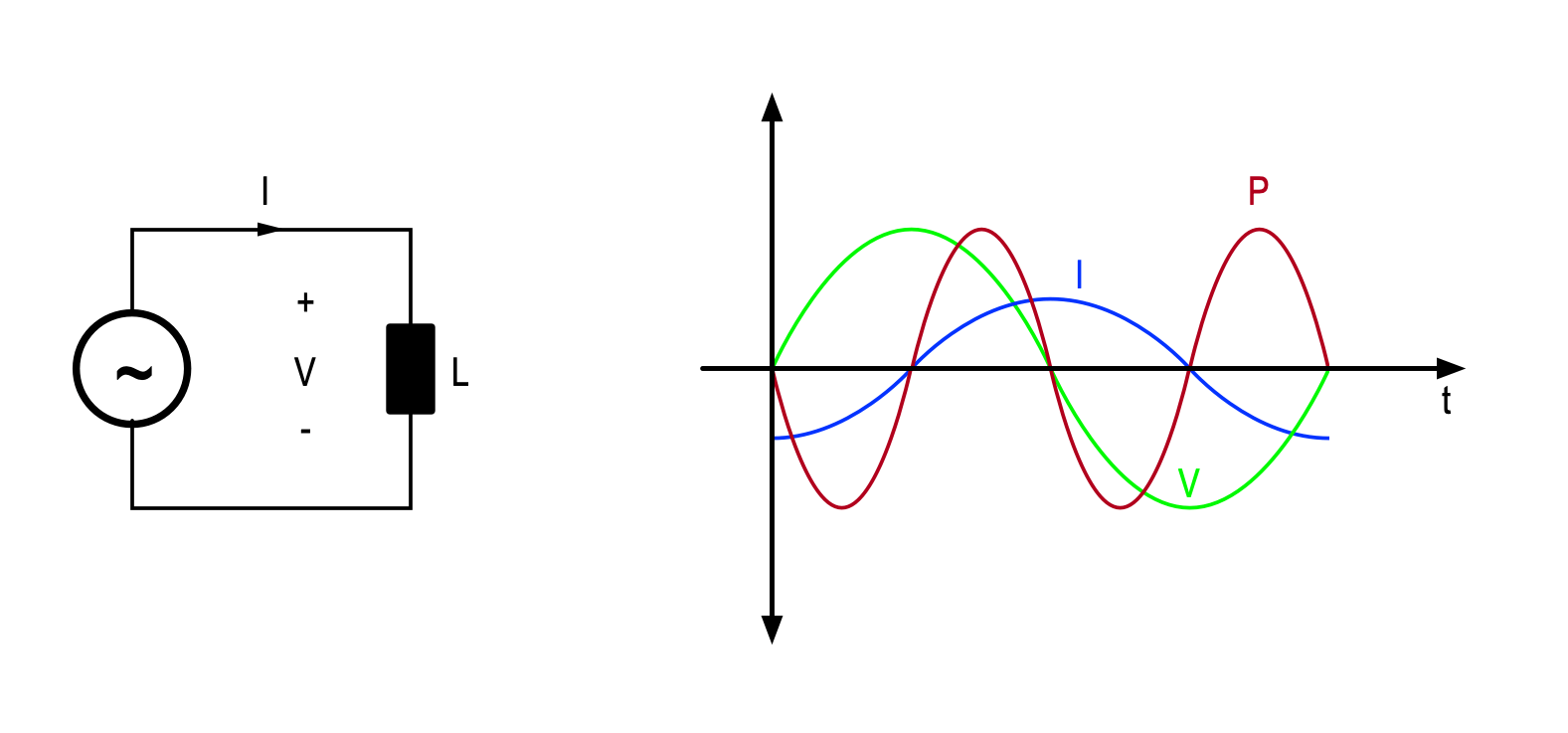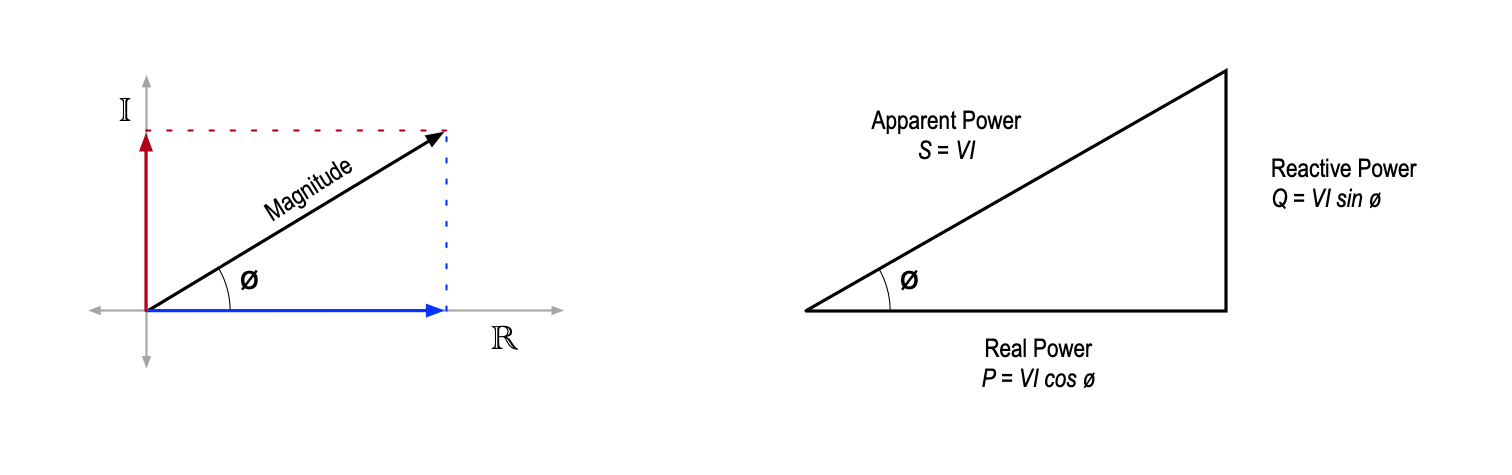# Real and Imaginary PowerWritten by

We all know that power is the product or voltage and current right? Well, this is true but not by any means the whole story. If we are going to be precise (and we are) we can say that at any instant the instantaneous power Pi = Vi × Ii. In DC circuits the average value of voltage and current is equal to the instantaneous value so the average power is the same as the instantaneous power yielding the familiar relationship P = V I .

For AC circuits the values of voltage and current are constantly changing. Take for example the resistive circuit of Figure 1.  As you would expect, the sinusoidal voltage across the resistor (green trace) results in a sinusoidal current with the same phase (blue trace). In the first half-cycle, both the voltage and current are positive so the instantaneous power will also be positive. In the second half-cycle, both are negative, so the resulting power is also positive.FIGURE 1. Q1 is held off via R1 and C1 until the ON button is pressed when power is applied to the microcontroller via Q1. Q2 is switched on immediately via GPIO1 to latch Q1 and this Q2 on. To turn the circuit off, the GPIO is driven low, turning off both MOSFETs.

I will spare you the math, but the resulting power waveform is also a sinusoid (red trace), but with twice the frequency and offset above zero. You can probably tell by inspection that the average power in the resistor will be P = ½ (Vpk Ipk).

Now what if we have a capacitive load such as in Figure 2. The voltage and current are both still sinusoidal, but now the current “leads” the voltage by 90°. This means the current peaks 90° before the voltage does. This time the power is positive in the first and third quarter-cycles, when the capacitor is charging, and negative in the second and fourth quarter-cycles when the capacitor is discharging.  The power waveform is identical to that in Figure 1 but shifted down, so it straddles the horizontal axis.FIGURE 2. This enhancement allows the one button to switch the circuit on and off. It turns on in the same way as Figure 1, but now GPIO2 can detect when the button is pressed again and initiate a turn off. This GPIO is configured as an input with an internal pull-up.

It’s pretty clear that the average power is now zero—weird, since the magnitudes of voltage and current are identical. The only thing that has changed is the phase relationship. Figure 3 shows that the same thing happens with a purely inductive load. In this case the current lags the voltage by 90°, but the average power is also zero. These kinds of loads are called reactive loads, since electrical energy is flowing in and out of the load each cycle, rather than just into the load as for a resistive load.FIGURE 3. This circuit has the same functionality as Figure 2 but requires only one GPIO pin. When powered up the internal pull-up is sufficient to turn Q2 on, latching the power on. When the microcontroller detects the button has been pressed, the software reconfigures the GPIO as an output and drives the pin low to switch off Q2 and therefore also Q1.

I’m going to try to explain the next bit without resorting to (literally) complex math. We need to think of the voltage and current not just in terms of their value or magnitude, as we do in DC circuits, but also in terms of their phase. We call this a vector quantity, and traditionally use bold font to distinguish them from magnitude only (or scalar) quantities. The left-hand side of Figure 4 shows that we can describe a vector as an arrow with a length corresponding to its magnitude and an angle from horizontal corresponding to its phase. We can equally describe the same vector by its projection on the horizontal and vertical axes as shown.FIGURE 4. The left-hand side shows that we can describe a vector as an arrow with a length corresponding to its magnitude and an angle from horizontal corresponding to its phase. The Power Triangle, shown on the right-hand side is commonly used to illustrate these terms.

The horizontal axis is the real (R) axis and the vertical axis is the imaginary (I) axis. The lengths of the real and imaginary projections are related to the magnitude of the vector cosine and sine of the phase angle respectively. You can probably see that if the phase angle is zero, the projection on the real axis is equal to the magnitude and the imaginary part is zero. If the phase angle was 90°, the projection on the real axis would be zero, and the imaginary part would be equal to the magnitude. If the angle is between the two, as in the figure, there will be a real and imaginary part to the quantity.

This is what is going on with our power. If the phase difference between the voltage and current is zero, the resulting power only has a real component, which we see as average power. If the voltage and current are out of phase by 90° as for a purely reactive load, the power will only have an imaginary component, and the average power will be zero.

Most loads in AC systems will have some resistive and some reactive component, so one number is not enough to describe power in these cases. This is why we need terms like Real Power, Reactive Power, Apparent Power and Power Factor.

The Power Triangle, shown on the right-hand side of Figure 4 is commonly used to illustrate these terms.  This is just a re-drawing of the left-hand side of Figure 4 specific to power.

The hypotenuse of the triangle corresponds to the magnitude of the power vector. This is the Apparent Power (S) and is given by S = |V||I|, measured in Volt-Amps (VA). The adjacent side of the triangle corresponds to the real axis and is the Real Power (P) given by P = V I cos ø, measured in Watts (W). This corresponds to the average power. Finally, the opposite side of the triangle corresponds to the imaginary axis and is the Reactive Power (Q) given by Q = V I sin ø, measured in Volt-Amps Reactive (VAR).

The quantity cos ø is the Power Factor (PF) and varies from one for a perfectly resistive load, to zero for a perfectly reactive load. Power companies would like all loads to be resistive (PF = 1) since they must size their transmission networks for the Apparent Power, but only Real Power does useful work at the point of consumption.

Unfortunately, many modern loads, particularly electric motors and switched-mode power supplies have very low power factor. Domestic customers usually pay only for Real Power, but many commercial customers are charged for Apparent Power, driving them to spend a lot of money trying to “correct” the power factor.

References

By. “Ditch The Switch: A Soft Latching Circuit Roundup.” Hackaday (blog), June 24, 2019. https://hackaday.com/2019/06/24/ditch-the-switch-a-soft-latching-circuit-roundup/.

Arshad, Areeba. “Soft Latch Switch Circuit.” Circuits DIY (blog), January 28, 2020. https://circuits-diy.com/soft-latch-switch-circuit/.

 Keep up-to-date with our FREE Weekly Newsletter! Don't miss out on upcoming issues of Circuit Cellar. Subscribe to Circuit Cellar Magazine Note: We’ve made the May 2020 issue of Circuit Cellar available as a free sample issue. In it, you’ll find a rich variety of the kinds of articles and information that exemplify a typical issue of the current magazine. Would you like to write for Circuit Cellar? We are always accepting articles/posts from the technical community. Get in touch with us and let's discuss your ideas.